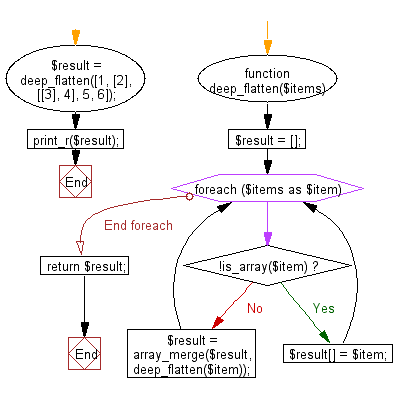﻿ PHP Exercise: Deep flatten an given array - w3resource

# PHP Exercises: Deep flatten an given array

## PHP: Exercise-79 with Solution

Write a PHP program to deep flatten an given array.

Sample Solution: -

PHP Code:

``````<?php
function deep_flatten(\$items)
{
\$result = [];
foreach (\$items as \$item) {
if (!is_array(\$item)) {
\$result[] = \$item;
} else {
\$result = array_merge(\$result, deep_flatten(\$item));
}
}
return \$result;
}
\$result = deep_flatten([1, , [, 4], 5, 6]);
print_r(\$result);

?>
```
```

Sample Output:

```Array
(
 => 1
 => 2
 => 3
 => 4
 => 5
 => 6
)```

Flowchart:PHP Code Editor:

Have another way to solve this solution? Contribute your code (and comments) through Disqus.

What is the difficulty level of this exercise?

Test your Programming skills with w3resource's quiz.

﻿

## PHP: Tips of the Day

Returns all elements in an array except for the first one

Example:

```<?php
function tips_tail(\$items)
{
return count(\$items) > 1 ? array_slice(\$items, 1) : \$items;
}

print_r(tips_tail([1, 5, 7]));
?>
```

Output:

```Array
(
 => 5
 => 7
)
```